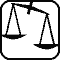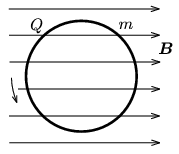Mathematical and Physical Journal
for High Schools
Issued by the MATFUND Foundation
 Already signed up? New to KöMaL?

# Exercises and problems in PhysicsSeptember 2003## Experimental problem

M. 244. Measure the water content as percentage of some fruits.

(6 points)## Theoretical problems

It is allowed to send solutions for any number of problems, but final scores of students of grades 9-12 are computed from the 5 best score in each month. Final scores of students of grades 1-8 are computed from the 3 best scores in each month.

P. 3632. Two identical boats are approaching the shore of a lake. The two rowers both pull themselves to the shore with the help of a rope. One of the ropes is fixed to a bitt on the shore, the end of the other is pulled by a man. Which boat lands first if all three men can pull with the same force?

(4 points)

P. 3633. There is a man of a mass of 100 kg sitting on a motorbike of a mass of 150 kg. The distance of the axles of the wheels (wheelbase) is 1.5 m. The common mass center of the man and the motorbike is at a 1 m height above the ground level, and at a 0.6 m distance from the vertical line going through the rear axle. What force is exerted on the rear wheel by the ground, when the motorbike starts off with an acceleration of a=2 m/s2? What acceleration is needed to lift the forward wheel? What friction coefficient can ensure this acceleration?

(4 points)

P. 3634. We immerse a slender, 1 m long glass pipe into water up to its half height, then we close the upper end by our finger. After this we slowly take the pipe out and turn it upwards (so the end closed by a finger gets to the bottom, and the water on top). How long is the air column stuck under the water if the pressure outside is 105 kPa?

(4 points)

P. 3635. In a vessel there is a compound of 14 g Nitrogen and 9 g Hydrogen gas at a temperature of 17 oC and a pressure of 100 kPa. What is the volume and the internal energy of the compound?

(3 points)

P. 3636. In a closed vessel of a volume of 4 l there is air of normal pressure and temperature and a solid body of a volume of 500 cm3 and a mass of 1 g. How much air is to be pumped into the vessel to make the body float?

(3 points)

P. 3637. There is ice of a temperature of 0 oC in a vacuum flask and water vapour of a temperature of 100 oC is loaded into it. What could the mass ratio of the ice and the vapour be to have only water in the flask after the thermal equilibrium is reached?

(4 points)

P. 3638. We connect the end points of a 40 Ohm slide rheostat to the outlets of a 12 V battery, then we connect a 20 Ohm consumer between one end of the rheostat and its slider.

a) In what position of the slider does the consumer get a voltage of 6 V?

b) In this case, what percentage of the battery power output is the power of the consumer?

c) What is the power of the consumer when the slider is in the middle?

(4 points)

P. 3639. A thin ring of mass m and electric charge Q rotates around an axis perpendicular to its plane and going through its center. The angular momentum of the ring is 7.5.10-4 kg.m2/s. The ring is in a homogeneous magnetic field of a field strength of 0.1 T and the lines of the magnetic induction are parallel with the plane of the ring. What torque is exerted on the ring? The specific charge (charge-mass ratio) of the ring is Q/m=10-5 C/kg.(4 points)

P. 3640. What kind of mirror of what focal length is it, in which at the distance of clear vision (25 cm) we can see our face in a 1.5 magnification? How far is our face from the mirror?

(4 points)

P. 3641. The mass of a neutron star is about the same as that of the Sun and its density is about the same as that of an atomic nucleus. How big is its radius? What is the acceleration of gravity on its surface?

(4 points)